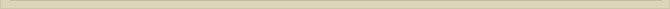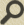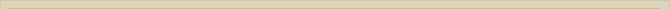## The proton and neutron mathematically

When you are interested in physics you must read “Unbelievable“!

The proton can be considered to emerge out of fusion of n+1 positrons and n electrons. The formula for the total energy of the proton becomes: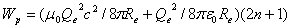Rp=Re/(2n+1)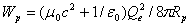When the energy of the proton is equally divided over magnetic and electrostatic energy the radius Rp is calculated with the mass Mp of the proton: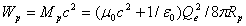The radius is calculated at Rp=1.535.10^-18 meter. This radius is a possible indication for the radius of the point-volume. A proton, in reality emerges out of a neutron that ejects an electron. Reversed, a neutron might emerge from the fusion of a proton and electron. The equations for proton en electron are: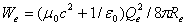When both particles fuse, the neutron emerges. Although the neutron is not charged, this does not mean the electrostatic energy of both particles is lost. The neutron has, like the proton and electron, two degrees of freedom to store energy. The neutron lacks the potential to store electrostatic energy. Instead it has the electromagnetic property to oscillate. When we fuse the proton and electron we get the formula: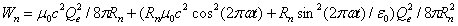The first part of this equation presents the magnetic spin energy of the neutron. The second part the oscillation energy. Assuming the energy is equally divided over both degrees of freedom we calculate Rn=1.533.10^-18 meter.

When an observer is situated in the neutron the observer is at rest with the ether under control/influence of the neutron. The observer spins with the neutron and can only observe the oscillation (second part Wn). When both charges +Qe and –Qe, in oscillation, overlap completely, the potential energy of the oscillation is nil and the magnetic/kinetic energy of the oscillation peaks. When the distance between both charges is maximal all oscillation energy is potential.

The electrostatic force between the two charges is calculated with the differential of the second part of equation Wn. The electrostatic force between both charges is: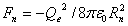Fn=-4.91.10^7 Newton

The positive and negative point-volume present each half of the energy/mass of the neutron. Both point-volumes have the force Fn (Fn=Fm) working. When we calculate the acceleration of each particle with a=2Fn/Mn, the classical way, we find that both particles move exact with the speed of light when they pass each other.

a= 2Fn/Mn     S=1/2Rn    T=(2S/a)^(1/2)      T=(Rn/a)^(1/2)     T=5.11.10^-27 time

is the distance each point-volume travels before they pass each other. The difference between the calculated speed of passage V=aT and c is so small that the difference is explained the margin errors of the mass of the neutron. The calculation fits exactly. The coincidence would be extreme, when the derived force accelerates the particles according to classical mechanics exactly to the speed of light c, when the proposed relation does not exist. The neutron is in a simple way explained means of the ether and classical mechanics.

When calculating the acceleration of both point-volumes in the neutron we stated that Fn was working on both sides. So we calculated the acceleration with a force equal to 2Fn. In the above we only accounted for de electrostatic force/energy and therefore we only incorporated half the energy of the neutron. We neglected the magnetic spin energy/force of the neutron.

Now we assume the observer is situated outside the neutron at rest with the surrounding ether. The observer sees now a neutral particle. The oscillation is too fast to observe. The spin of the neutron induces a current in the surrounding ether. The induced spin current in the ether surrounding the neutron is not in balance with the ether outside the neutron. The magnetic force, the rejection the surrounding ether of this current, is derived differentiation of the first part of the equation for the energyWn of the neutron.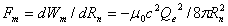The magnetic force is equal to the electrostatic force (Fm=Fn). Calculating the oscillating with 2Fn is justified because the total force is the sum of the electrostatic force Fn plus the magnetic force Fm.

When the neutron oscillates over a distance Rn it has to “push” away the surrounding ether. The total energy of the neutron can also be stated as: Wn=Rn(Fm+Fn).

Next chapter: The photon and the constant of Planck (h)